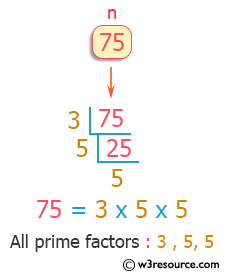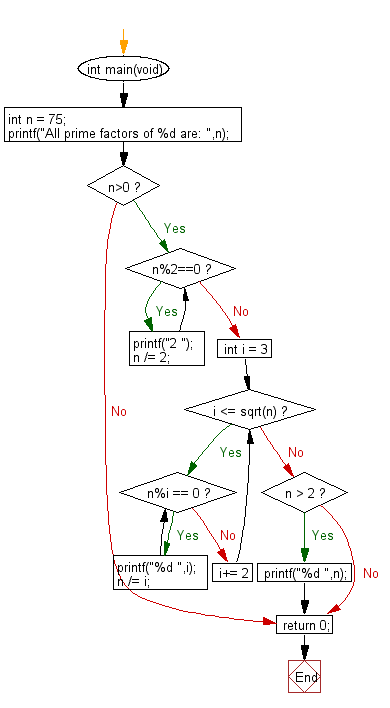﻿ C Program: Print all prime factors of a given number - w3resource

# C Exercises: Print all prime factors of a given number

## C Programming Mathematics: Exercise-25 with Solution

Write a C program to print all prime factors of a given number.

Example:
Input: n = 75
Output: All prime factors of 75 are: 3 5 5

Pictorial Presentation:Sample Solution:

C Code:

``````#include <stdio.h>
#include <stdlib.h>

int main(void)
{
//int n = 8;
int n = 75;
// int n = 18;
printf("All prime factors of %d are: ",n);
if (n>0)
{
while (n%2==0)
{
printf("2 ");
n /= 2;
}

for (int i = 3; i <= sqrt(n); i+= 2)
{
while (n%i == 0)
{
printf("%d ",i);
n /= i;
}
}
if (n > 2)
printf("%d ",n);
}
return 0;
}
```
```

Sample Output:

```All prime factors of 75 are: 3 5 5
```

Flowchart:C Programming Code Editor:

Improve this sample solution and post your code through Disqus.

What is the difficulty level of this exercise?

Test your Programming skills with w3resource's quiz.

﻿